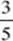﻿ ﻿Answers and Explanations - Polynomial Functions - Functions - REVIEW OF MAJOR TOPICS - SAT SUBJECT TEST MATH LEVEL 2

## PART 2## REVIEW OF MAJOR TOPICS## CHAPTER 1Functions

###1.2 Polynomial FunctionsLinear Functions

1. (C)2. (B). The slope is.

3. (A). The slope of the given line is. The slope of a perpendicular line is.

4. (C) The slope of the line is, so the point-slope equation is. Solve for y to get. The y-intercept of the line is.

5. (C) The slope of the segment is. Therefore, the slope of a perpendicular line is –3. The midpoint of the segment is. Therefore, the point-slope equation is. In general form, this equation is 3x + y – 2 = 0.

* 6. (D) Length =7. (C). Therefore, the slope of a parallel line.

* 8. (C) The slope of the first line is, and the slope of the second line is. To be perpendicular,.

1. (B) The x coordinate of the vertex isand the y coordinate is y = 2(–1)2 + 4(–1) – 5 = –7. Hence the vertex is the point (–1,–7).

2. (C) Find the vertex:and y = 5 – 4(–2) – (–2)2 = 9. Since a = –1 < 0 the parabola opens down, so the range is {y : y9}.

3. (B) The x coordinate of the vertex is. Thus, the equation of the axis of symmetry is.

4. (E) 2x2 + x – 6 = (2x – 3)(x + 2) = 0. The zeros areand –2.

5. (D) Sum of.

6. (E) From the discriminant b2 – 4ac = 4 – 4 · 1 · 3 = –8 < 0.

* 7. (B) The equation of a vertical parabola with its vertex at the origin has the form y = ax2 . Substitute (7,7) for x and y to find. When y = 6, x2 = 42. Therefore,, and the segment =.

Higher-Degree Polynomial Functions

1. (A) Since the degree of the polynomial is an even number, both ends of the graph go off in the same direction. Since P(x) increases without bound as x increases, P(x) also increases without bound as x decreases.

2. (C) Since the exponents are all odd, and there is no constant term, III is the only odd function.

3. (E) Rational roots have the form, where p is a factor of 12 and q is a factor of 2.. The total is 16.

* 4. (A) Since x – 1 is a factor, P(1) = 13 – 3 · 12 + 2 · 1 – 4b = 0. Therefore, b = 0.

* 5. (D) Substitute 3 for x set equal to zero and solve for K.

6. (D) 1 – i is also a root. To find the equation, multiply (x + 1)[x – (1 + i)][x – (1 – i)], which are the factors that produced the three roots.

Inequalities

1. (C) 3x2x – 2 = (3x + 2)(x – 1) = 0 whenor 1. Numbers between these satisfy the original inequality.

* 2. (C) Graph the function, and determine that the three zeros are –1.90, –0.87, and 1.58. The parts of the graph that are above the x-axis have x-coordinates between –1.90 and –0.87 and are larger than 1.58.

3. (C) x2 – 16x + 48 = (x – 4)(x – 12) = 0, when x = 4 or 12. Numbers between these satisfy the original inequality.

﻿